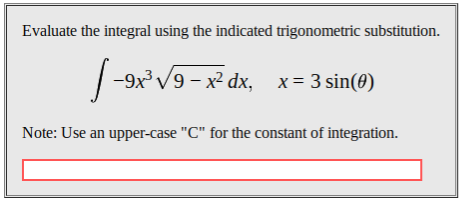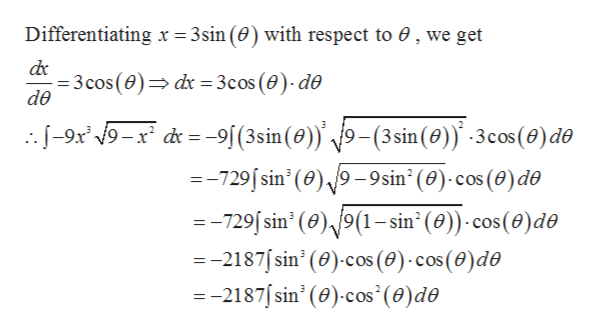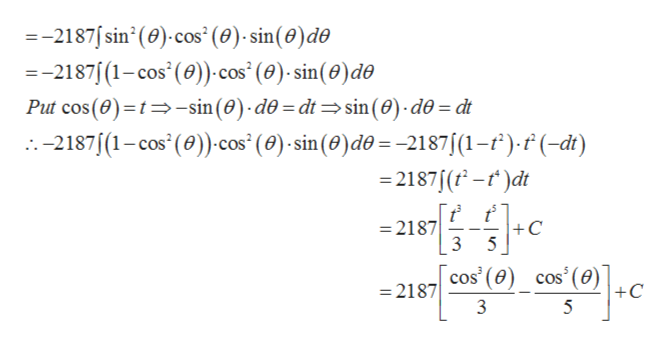# Evaluate the integral using the indicated trigonometric substitution9x3 V9 x2 dx,x 3 sin(0)Note: Use an upper-case "C" for the constant of integration.

Question
3 viewshelp_outlineImage TranscriptioncloseEvaluate the integral using the indicated trigonometric substitution 9x3 V9 x2 dx, x 3 sin(0) Note: Use an upper-case "C" for the constant of integration. fullscreen
check_circle

Step 1

Given,

Step 2

On simplificatonhelp_outlineImage TranscriptioncloseDifferentiating x = 3sin (0) with respect to 0, we get 3cos(0) dx = 3cos (0)- de de --9x 9-x d=-9[(3sin (0)) 9-(3sin (0) -3cos(e)de =-729/sin' (0)/9-9sin2(0) cos (0)de =-729 sin' (0),/9(1-sin2 (0)) cos (0)de =-2187sin' (0) cos (0)-cos (0)de --2187/sin' (e) cos' (0) de fullscreen
Step 3

Now

...help_outlineImage Transcriptionclose=-2187sin' (0) cos (0)- sin(0)de --2187 (1-cos (0)) cos (0)-sin(0) de Put cos(e) t-sin(0) d0 = dt sin (0) d0= dt -2187 (1-cos' (0))cos' (e) sin (0)do= -2187/(1-f).f (-dt) -2187 (-)dt =2187 3 +C 5 cos' (0) cos (e) +C 2187 5 fullscreen

### Want to see the full answer?

See Solution

#### Want to see this answer and more?

Solutions are written by subject experts who are available 24/7. Questions are typically answered within 1 hour.*

See Solution
*Response times may vary by subject and question.
Tagged in

### Integration